# 12-240/Classnotes for Tuesday September 11

(diff) ← Older revision | Latest revision (diff) | Newer revision → (diff)

In this course, we will be focusing on both a practical side and a theoretical side.

## Practical Side

1. Solving complicated systems of equations, such as: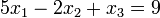$5x_1 - 2x_2 + x_3 = 9\!$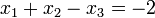$x_1 + x_2 - x_3 = -2\!$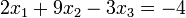$2x_1 + 9x_2 - 3x_3 = -4\!$

2. We can turn the above into a matrix!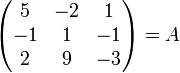$\begin{pmatrix} 5 & -2 & 1 \\ -1 & 1 & -1 \\ 2 & 9 & -3 \end{pmatrix} = A$

## Theory Side

3. "The world doesn't come with coordinates." We will learn to do all of this in a coordinate-free way.

4. We'll learn to do all of this over other sets of numbers and fields.

## Hidden Agenda

5. We'll learn the process of pure mathematics by doing it. We'll learn about:

• Abstraction
• Generalization
• Definitions
• Theorems
• Proofs
• Notation
• Logic

A number system has specific properties of the real numbers.

## Real Numbers

A set,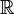$\mathbb{R}\!$, with:

• Two binary operations, addition and multiplication.
• Two special elements, 0 and 1.

The real numbers have some special properties:

### Commutative Laws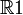$\mathbb{R}1$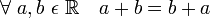$\forall\ a, b\ \epsilon\ \mathbb{R} \quad a+b = b+a\!$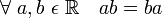$\forall\ a, b\ \epsilon\ \mathbb{R} \quad ab = ba\!$

### Associative Laws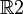$\mathbb{R}2$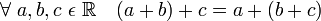$\forall\ a, b, c\ \epsilon\ \mathbb{R} \quad (a + b) + c = a + (b + c)\!$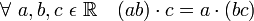$\forall\ a, b, c\ \epsilon\ \mathbb{R} \quad (ab) \cdot c = a \cdot (bc)\!$

### Existence of "Units"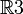$\mathbb{R}3$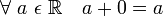$\forall\ a\ \epsilon\ \mathbb{R} \quad a + 0 = a\!$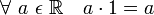$\forall\ a\ \epsilon\ \mathbb{R} \quad a \cdot 1 = a\!$

### Existence of Negatives/Inverses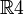$\mathbb{R}4$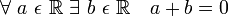$\forall\ a\ \epsilon\ \mathbb{R}\ \exists\ b\ \epsilon\ \mathbb{R} \quad a + b = 0\!$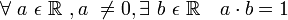$\forall\ a\ \epsilon\ \mathbb{R}\ , a\ \neq 0 , \exists\ b\ \epsilon\ \mathbb{R} \quad a \cdot b = 1\!$

### Distributive Law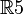$\mathbb{R}5$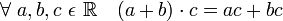$\forall\ a, b, c\ \epsilon\ \mathbb{R} \quad (a+b) \cdot c = ac + bc\!$

#### An example of a property that follows from the earlier ones: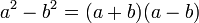$a^2 - b^2 = (a + b)(a - b)\!$

We can define subtraction and squaring from the properties covered above.

#### An example of a property that does not follow from the earlier ones:

The existence of square roots: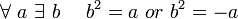$\forall\ a\ \exists\ b\ \quad b^2 = a\ or\ b^2 = -a\!$

We can construct a set that has all of the 5 properties described above, but for which this property does not follow.

This set is the rational numbers.

There is a rational number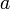$a\!$ where there is no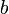$b$ in the set.

This is because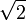$\sqrt{2}$ is irrational.

## Fields

The properties we have been discussing aren't restricted to only the real numbers.

They are also properties of:

• Rational numbers
• Complex numbers
• Others

Let's construct an abstract universe where these properties hold.

Definition: Field

• A field is a set,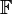$\mathbb{F}$, with:
• Two binary operations, addition and multiplication.
• Two special elements, 0 and 1, where 0 does not equal 1.
• All of the above mentioned properties hold.

Now, instead of speaking of$\mathbb{R}1,\ \mathbb{R}2,\ \mathbb{R}3,\ \mathbb{R}4,\ \mathbb{R}5$, we can speak of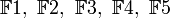$\mathbb{F}1,\ \mathbb{F}2,\ \mathbb{F}3,\ \mathbb{F}4,\ \mathbb{F}5$.

We have abstracted!

## Examples of Fields

• Take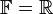$\mathbb{F} = \mathbb{R}$

• Take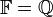$\mathbb{F} = \mathbb{Q}$ (Rational numbers)

• The complex numbers.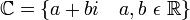$\mathbb{C} = \lbrace a + bi \quad a, b\ \epsilon\ \mathbb{R} \rbrace$

The above fields have an infinite number of elements. We can also have finite fields:

•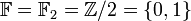$\mathbb{F} = \mathbb{F}_2 = \mathbb{Z}/2 = \lbrace 0, 1 \rbrace$
• There are only 2 elements.
• You can think of 0 as even and 1 as odd, which will help you construct the tables below.
• You can also think of the results below as the remainder of the operations when divided by 2. (mod 2)
+ 0 1
0 0 1
1 1 0
x 0 1
0 0 0
1 0 1
• Question: Are addition and multiplication defined here only arbitrary? Can we define many other ways to add or multiply, for a set, as long as the result satisfies F1-5 to show that F is indeed a field?
• Answer by Drorbn 16:51, 13 September 2012 (EDT): A "field" is a set with two operations and 0 and 1 so that some properties hold. In principle, the same set can be made into a field in many different ways - by choosing different operations (so long as they satisfy F1-5). In practice though, there is essentially only one field with 5 elements (but I the word "essentially" here requires an explanation). Many other sets can be considered as fields in many "genuinely different" ways, depending in how you choose the operations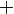$+$ and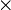$\times$.
•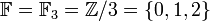$\mathbb{F} = \mathbb{F}_3 = \mathbb{Z}/3 = \lbrace 0, 1, 2 \rbrace$
+ 0 1 2
0 0 1 2
1 1 2 0
2 2 0 1
x 0 1 2
0 0 0 0
1 0 1 2
2 0 2 1

•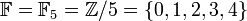$\mathbb{F} = \mathbb{F}_5 = \mathbb{Z}/5 = \lbrace 0, 1, 2, 3, 4 \rbrace$
• Not going to bother making the tables here.

•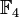$\mathbb{F}_4$ is not a field.
• It does not have the property$\mathbb{R}4$.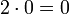$2 \cdot 0 = 0$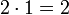$2 \cdot 1 = 2$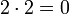$2 \cdot 2 = 0$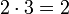$2 \cdot 3 = 2$
We never got a 1.

• If the subscript is a prime number, it is a field.

Theorem:

1.

Let F be a field.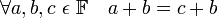$\forall a, b, c\ \epsilon\ \mathbb{F} \quad a+b = c+b$
"Cancellation Lemma"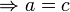$\Rightarrow a = c$

2.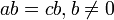$ab = cb, b \ne 0$$\Rightarrow a = c$

We'll cover 3-11 next class!

Proof of 1:

Let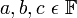$a, b, c\ \epsilon\ \mathbb{F}$
by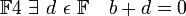$\mathbb{F} 4\ \exists\ d\ \epsilon\ \mathbb{F} \quad b+d = 0$
so with this d,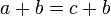$a+b = c+b\!$
and so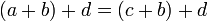$(a+b)+d = (c+b)+d\!$
so by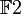$\mathbb{F} 2$,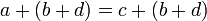$a+(b+d) = c+(b+d)\!$
so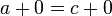$a+0 = c+0\!$
so by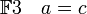$\mathbb{F} 3 \quad a = c\!$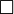$\Box$

## Scanned Notes by Sina.zoghi

Sina.zoghi - Thanks for improving on the previously-uploaded scans - though there is still too much "white space" around each page. It is probably not worth your while to fix it for these scans, but it is something to keep in mind for later ones. Drorbn 10:50, 13 September 2012 (EDT)

## Lecture notes upload by Starash

Another upload of lecture 1 notes.